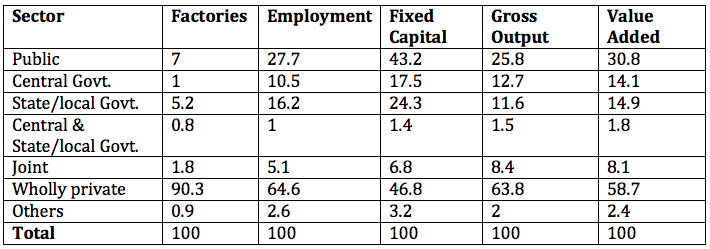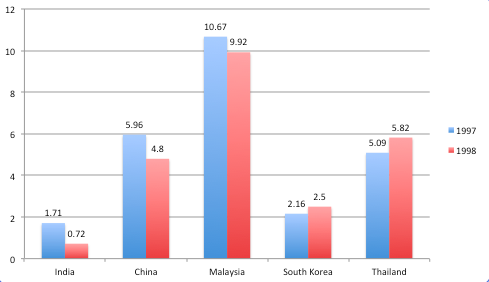# CAT 2000

Instructions

For the following questions answer them individually

Question 11

# Consider three real numbers, X, Y, and Z. Is Z the smallest of these numbers?A. X is greater than at least one of Y and Z. B. Y is greater than at least one of X and Z.Question 12

# Let X be a real number. Is the modulus of X necessarily less than 3?A. X(X+3)<0B. X(X-3)>0Instructions

Directions for the next 5 questions: Answer these questions based on the data provided in the table below: Factory Sector by Type of Ownership. All figures in the table are in percent of the total for the corresponding column.Question 13

# Suppose the average employment level is 60 per factory. The average employment in wholly private” factories is approximately:Question 14

# Among the firms in different sectors, value added per employee is highest in:Question 15

# Capital productivity is defined as the gross output value per rupee of fixed capital. The three sectors with the higher capital productivity, arranged in descending order are:Question 16

# A sector is considered ‘pareto efficient’ if its value added per employee and its value added per rupee of fixed capital is higher than those of all other sectors. Based on the table data, the pareto efficient sector is:Question 17

# The total value added in all sectors is estimated at Rs. 14,000 crores. Suppose that the number of firms in the joint sector is 2700. The average value added per factory, in Rs. crores, in the central govt. is:Instructions

Directions for the next 4 questions: Answer these questions based on the data presented in the figure below. FEI for a country in a year, is the ratio (expressed as a percentage) of its foreign equity inflows to its GDP. The following figure displays the FEIs for select Asian countries for the years 1997 and 1998.Question 18

# The country with the highest percentage change in FEI in 1998 relative to its FEI in 1997, is:Question 19

# Based on the data provided, it can be concluded thatQuestion 20

# It is known that China’s GDP in 1998 was 7% higher than its value in 1997, while India’s GDP drew by 2% during the same period. The GDP of South Korea, on the other hand, fell by 5%.Which of the following statements is/are true? Foreign equity inflows to China were higher in 1998 than in 1997. Foreign equity inflows to China were lower in 1998 than in 1997. Foreign equity inflows to India were higher in 1998 than in 1997. Foreign equity inflows to South Korea decreased in 1998 relative to 1997. Foreign equity inflows to South Korea increased in 1998 relative to 1997,OR